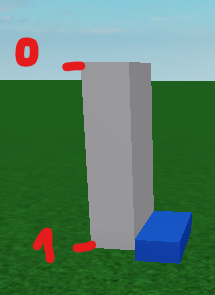# Getting a part's relative position in %

Hi! I’ve spent like an hour trying to figure this out. So I have 1 part A, and I want to know it’s y position relative to the other part B but that number should be between 0 and 1. If the part A is at the bottom of the part B (again, only height matters, so the Y axis), I want to get the number 1. If its at the top, I want to the get the number 0. If it’s in the middle, I want the number 0.5. You get what I mean. Could somene explain to me, maybe even give a formula, how I can solve this issue?

Image of what I want to achieve, the blue part being the one that’s moved.`alpha = (y_top - y_blue) / (y_top - y_bottom)`

An example, with the top as y=7, the bottom as y=3, and the blue part at y=4:
`(7 - 4) / (7 - 3) = 0.75`

Simply implement that in Lua, fetching the Y position by using Part.Position.Y, where Part is the part you’re interested in.

You may want to clamp the values with math.clamp() to avoid values less than 0 and greater than 1 if the blue part is beyond the bounds.

You can get the top and bottom of the grey part programmatically using the grey part’s Y position + half of its size in the Y axis.

1 Like